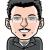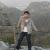## 15个值得开发人员关注的jQuery开发技巧和心得

### 1. 尽量使用最新版本的jQuery类库

jQuery项目中使用了大量的创新。最好的方法来提高性能就是使用最新版本的jQuery。每一个新的版本都包含了优化的bug修复。对我们来说唯一要干的就是修改tag，何乐而不为呢？

```<!-- Include a specific version of jQuery -->

### 2. 使用简单的选择器

```\$('li[data-selected="true"] a')	// Fancy, but slow
\$('li.selected a')	// Better
\$('#elem')	// Best```

```var buttons = \$('#navigation a.button');  // Some prefer prefixing their jQuery variables with \$:

```\$('a.button:animated');	// Does not use querySelectorAll()
\$('a.button').filter(':animated');	// Uses it```

### 3. 数组方式使用jQuery对象

```// Selecting all the navigation buttons:

// We can loop though the collection:
for(var i=0;i<buttons.length;i++){
console.log(buttons[i]);	// A DOM element, not a jQuery object
}

// We can even slice it:
var firstFour = buttons.slice(0,4);```

```if(buttons){	// This is always true
// Do something
}

if(buttons.length){ // True only if buttons contains elements
// Do something
}```

### 4. 选择器属性

jQuery提供了一个属性，这个属性显示了用来做链式的选择器。

```\$('#container li:first-child').selector    // #container li:first-child
\$('#container li').filter(':first-child').selector    // #container li.filter(:first-child)```

### 5. 创建一个空的jQuery对象

```var container = \$([]);

### 6. 选择一个随机元素

```(function(\$){
var random = 0;

\$.expr[':'].random = function(a, i, m, r) {
if (i == 0) {
random = Math.floor(Math.random() * r.length);
}
return i == random;
};

})(jQuery);

// This is how you use it:

### 7. 使用CSS Hooks

CSS hooks API是提供开发人员得到和设置特定的CSS数值的方法。使用它，你可以隐藏浏览器特定的执行并且使用一个统一的界面来存取特定的属性。、

```\$.cssHooks['borderRadius'] = {
get: function(elem, computed, extra){
// Depending on the browser, read the value of
},
set: function(elem, value){
// Set the appropriate CSS3 property
}
};

// Use it without worrying which property the browser actually understands:

### 8. 使用自定义的删除方法

```\$.easing.easeInOutQuad = function (x, t, b, c, d) {
if ((t/=d/2) < 1) return c/2*t*t + b;
return -c/2 * ((--t)*(t-2) - 1) + b;
};

// To use it:

### 9. \$.proxy()

```<div id="panel" style="display:none">
<button>Close</button>
</div>```

```\$('#panel').fadeIn(function(){
// this points to #panel
\$('#panel button').click(function(){
// this points to the button
});
});```

```\$('#panel').fadeIn(function(){
// Using \$.proxy to bind this:

\$('#panel button').click(\$.proxy(function(){
// this points to #panel
},this));
});```

### 10. 判断页面是否太过复杂

`console.log( \$('*').length );`

### 11. 将你的代码转化成jQuery插件

```(function(\$){
\$.fn.yourPluginName = function(){
return this;
};
})(jQuery);```

12.  设置全局AJAX为缺省

```// ajaxSetup is useful for setting general defaults:
\$.ajaxSetup({
url            : '/ajax/',
dataType    : 'json'
});

\$.ajaxStart(function(){
showIndicator();
disableButtons();
});

\$.ajaxComplete(function(){
hideIndicator();
enableButtons();
});

/*
// Additional methods you can use:
\$.ajaxStop();
\$.ajaxError();
\$.ajaxSuccess();
\$.ajaxSend();
*/```

### 13. 在动画中使用delay()方法

```// This is wrong:
\$('#elem').animate({width:200},function(){
setTimeout(function(){
\$('#elem').animate({marginTop:100});
},2000);
});

// Do it like this:
\$('#elem').animate({width:200}).delay(2000).animate({marginTop:100});```

jQuery动画帮了我们大忙，否则我们得自己处理一堆的细节，设置timtout，处理属性值，跟踪动画变化等等。

### 14. 合理利用HTML5的Data属性

HTML5的data属性可以帮助我们插入数据。特别合适前后端的数据交换。jQuery近来发布的data()方法，可以有效的利用HTML5的属性，来自动得到数据。下面是个例子：

```<div id="d1" data-role="page" data-last-value="43" data-hidden="true"
data-options='{"name":"John"}'>
</div>```

```\$("#d1").data("role");			// "page"
\$("#d1").data("lastValue");		// 43
\$("#d1").data("hidden");		// true;
\$("#d1").data("options").name;	// "John";```

data()的jQuery文档：data() in the jQuery docs

### 15. 本地存储和jQuery

`localStorage.someData = "This is going to be saved across page refreshes and browser restarts";`

### 最新评论（9）#### 引用来自“宋浩”的评论h

http://www.heronote.com/files/jQuery.htm